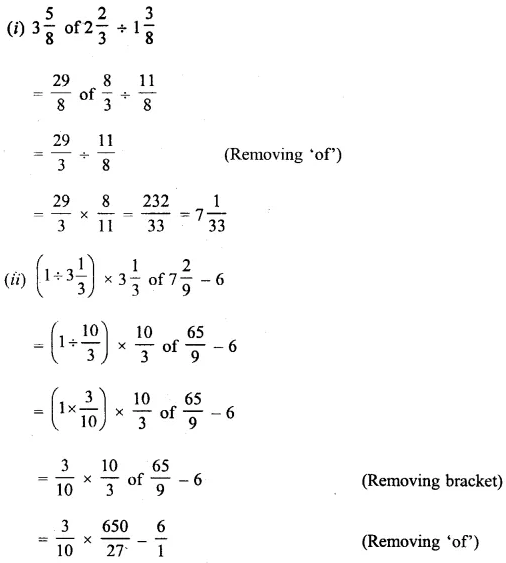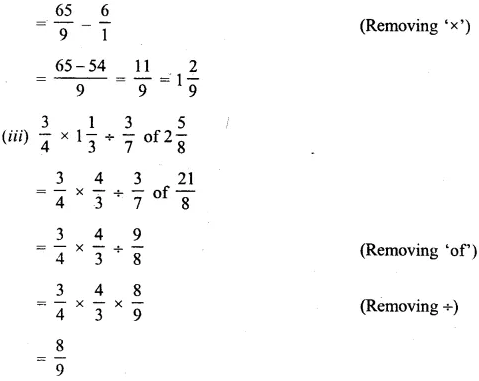# Selina Concise Mathematics Class 6 ICSE Solutions Chapter 14 Fractions

## Selina Concise Mathematics Class 6 ICSE Solutions Chapter 14 Fractions

Selina Publishers Concise Mathematics Class 6 ICSE Solutions Chapter 14 Fractions

### Fractions Exercise 14A – Selina Concise Mathematics Class 6 ICSE Solutions

Question 1.
For each expression, given below, write a fraction :
(i) 2 out of 7 = ………..
(ii) 5 out of 17 = ………..
(iii) three-fifths = ………
Solution: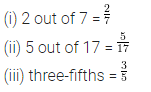Question 2.
Fill in the blanks :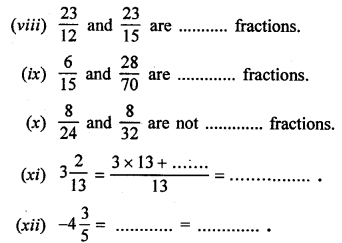Solution: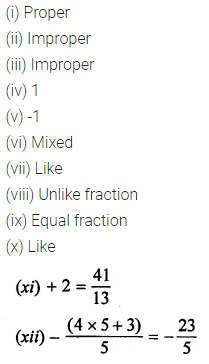Question 3.
From the following fractions, separate :
(i) Proper fractions
(ii)Improper fractions :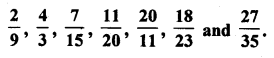Solution: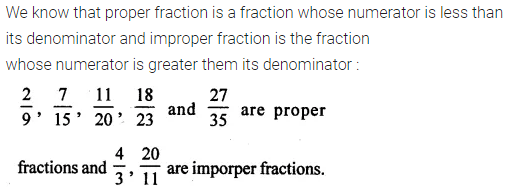Question 4.
Change the following mixed fractions to improper fractions :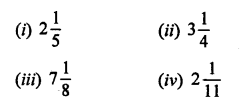Solution:Question 5.
Change the following improper fractions to mixed fractions :Solution:Question 6.
Change the following groups of fractions to like fractions :Solution: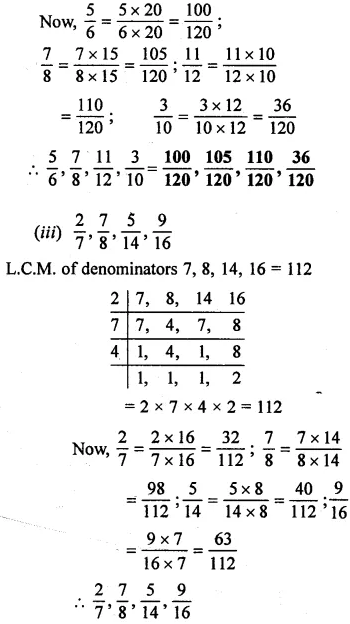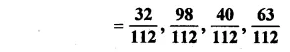### Fractions Exercise 14B – Selina Concise Mathematics Class 6 ICSE Solutions

Question 1.
Reduce the given fractions to their lowest terms :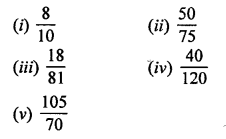Solution: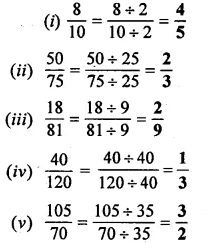Question 2.
State, whether true or false?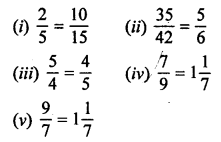Solution: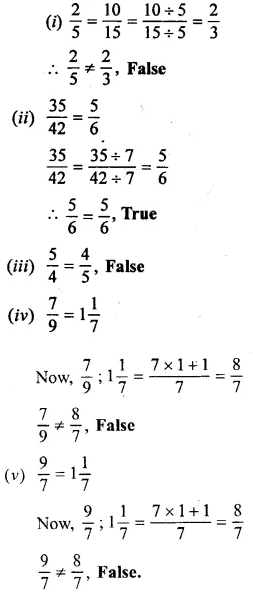Question 3.
Which fraction is greater?Solution: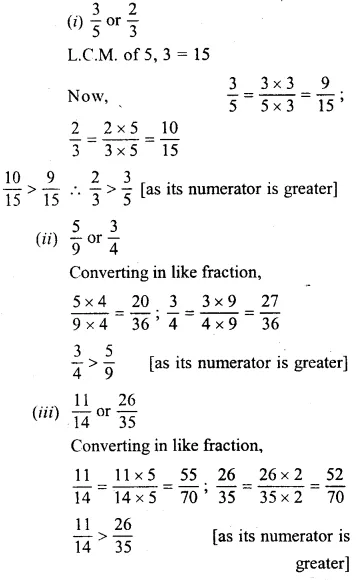Question 4.
Which fraction is smaller ?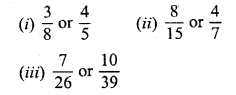Solution:Question 5.
Arrange the given fractions in descending order of magnitude :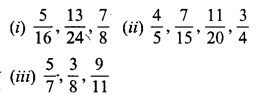Solution: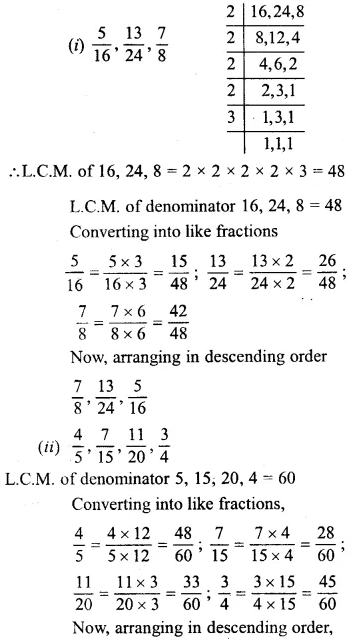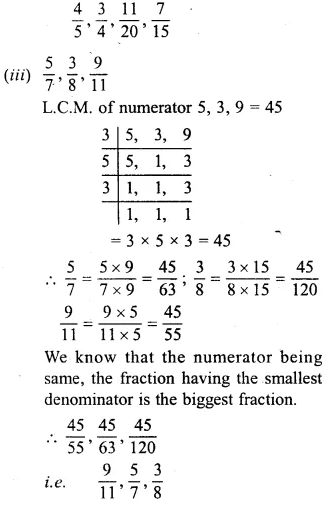Question 6.
Arrange the given fractions in ascending order of magnitude :Solution: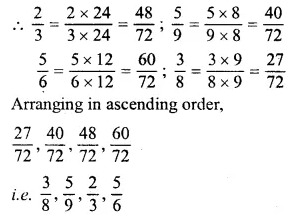Question 7.
I bought one dozen bananas and ate five of them. What fraction of the total number of bananas was left ?
Solution:Question 8.
Insert the symbol ‘=’ or ‘>’ or ‘<’ between each of the pairs of fractions, given below :Solution:Question 9.
Out of 50 identical articles, 36 are broken. Find the fraction of :
(i) The total number of articles and the articles broken.
(ii) The remaining articles and total number of articles.
Solution:### Fractions Exercise 14C – Selina Concise Mathematics Class 6 ICSE Solutions

Question 1.Solution: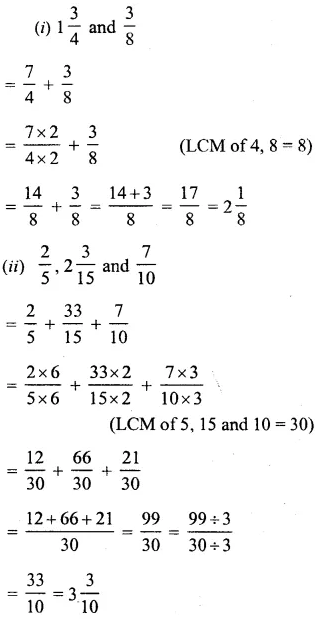Question 2.
Simplify :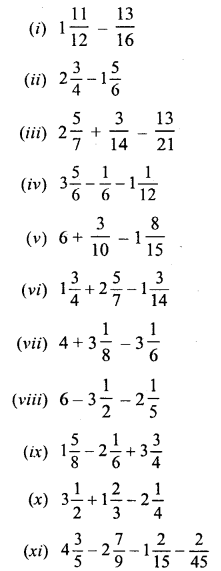Solution: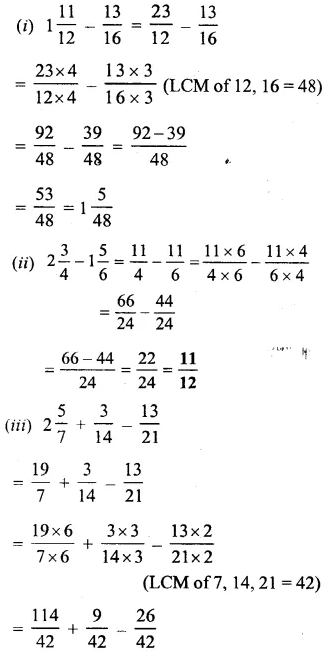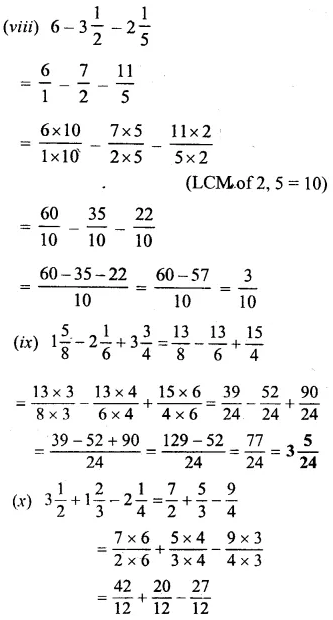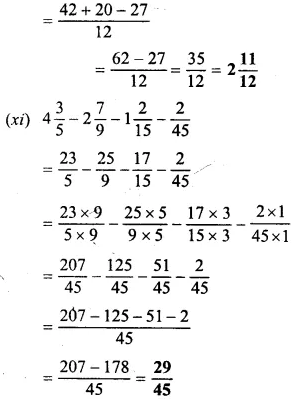### Fractions Exercise 14D – Selina Concise Mathematics Class 6 ICSE Solutions

Point to Remember :
BODMAS :- While simplifying an expressions we can involve six operation in following orders.
B Stands for “BRACKET”
O Stands for “OF”
D Stands for “DIVISION”
M Stands for “MULTIPLICATION”
S Stands for “SUBTRACTION”

Question 1.
Simplify :Solution: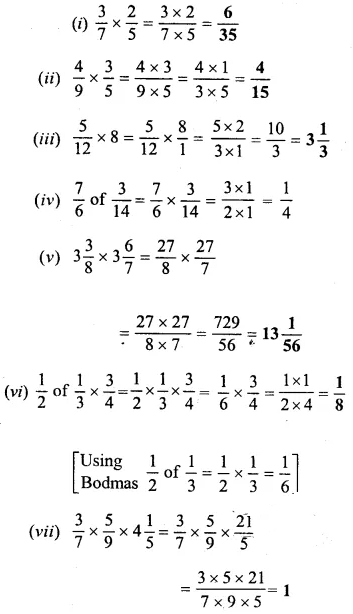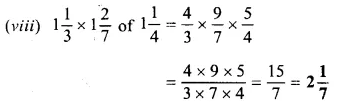Question 2.
Simplify :Solution: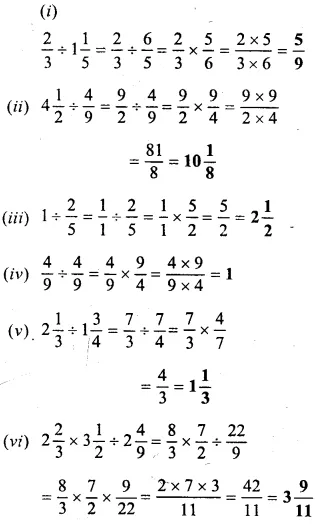Question 3.
Simplify :Solution: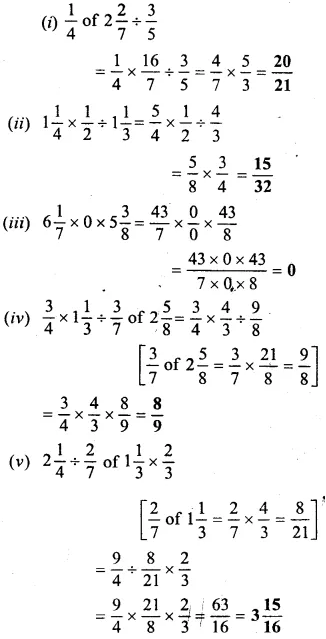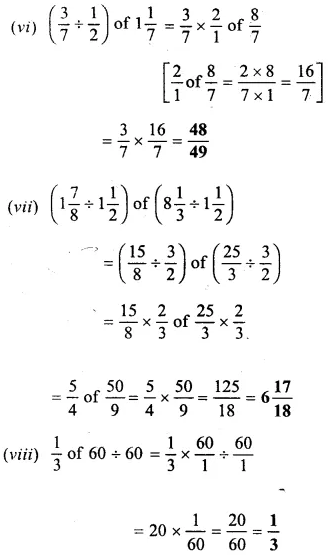Question 4.
Simplify :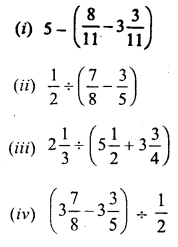Solution: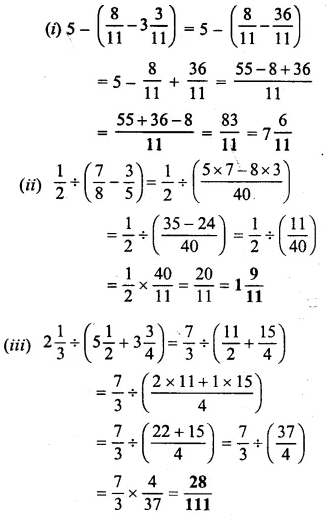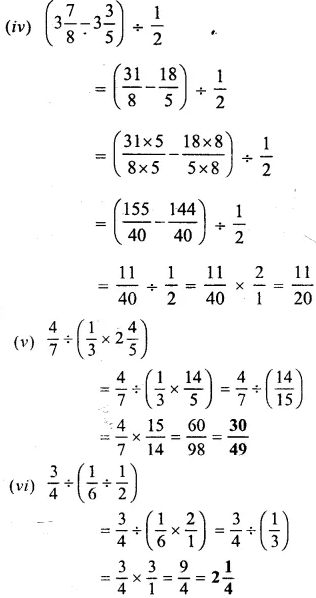Question 5.
Simplify :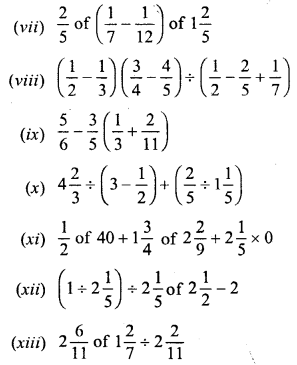Solution: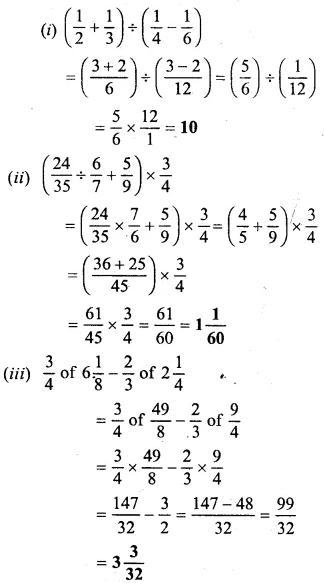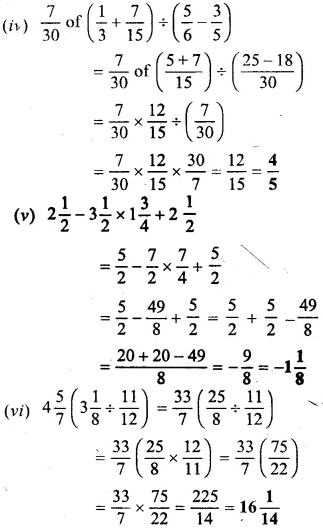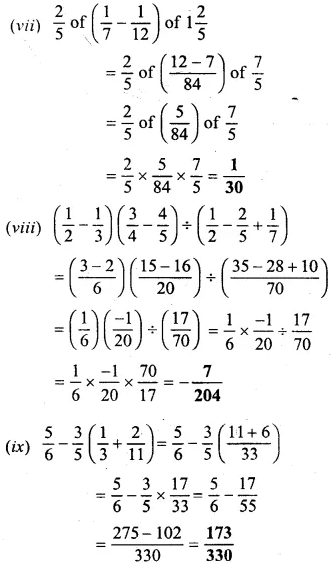### Fractions Exercise 14E – Selina Concise Mathematics Class 6 ICSE Solutions

Question 1.
From a rope of 10$$\frac { 1 }{ 2 }$$ m long, 4$$\frac { 5 }{ 8 }$$ m is cut off. Find the length of the remaining rope.
Solution:Question 2.
A piece of cloth is 5 metre long. After washing, it shrinks by $$\frac { 1 }{ 25 }$$ of its length. What is the length of the cloth after washing?
Solution: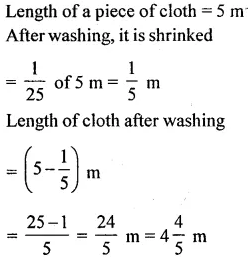Question 3.
I bought wheat worth Rs. 12$$\frac { 1 }{ 2 }$$, rice worth Rs. 25$$\frac { 3 }{ 4 }$$ and vegetables worth Rs. 10$$\frac { 1 }{ 4 }$$. If I gave a hundred-rupee note to the shopkeeper ; how much did he return to me
Solution: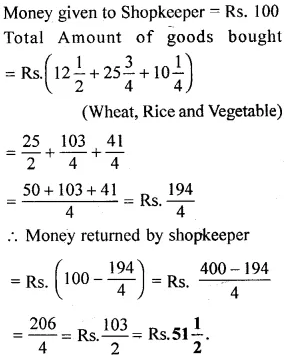Question 4.
Out of 500 oranges in a box, $$\frac { 3 }{ 25 }$$ are rotten and $$\frac { 1 }{ 5 }$$ are kept for some guests. How many oranges are left in the box?
Solution:Question 5.
An ornament piece is made of gold and copper. Its total weight is 96g. If $$\frac { 1 }{ 12 }$$ of the ornament hi copper, find the weight of gold in it.
Solution: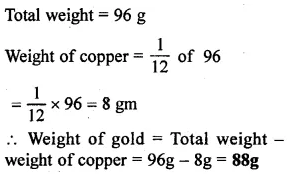Question 6.
A girl did half of some work on Monday and one-third of it on Tuesday. How much will she have to do on Wednesday in order to complete the work?
Solution: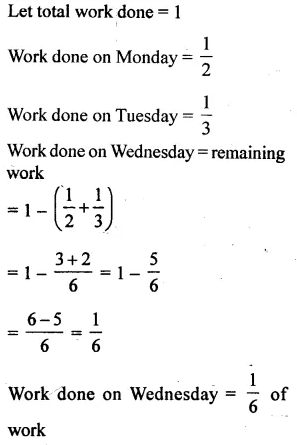Question 7.
A man spends $$\frac { 3 }{ 8 }$$ of his money and 8 still has Rs. 720 left with him. How much money did he have at first?
Solution:Question 8.
In a school, $$\frac { 4 }{ 5 }$$ of the students are boys, and the number of girls is 100. Find the number of boys.
Solution: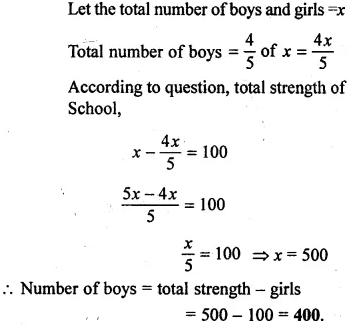Question 9.
After finishing $$\frac { 3 }{ 4 }$$ of my journey, I find that 12 km of my journey is covered. How much distance is still left to be covered?
Solution: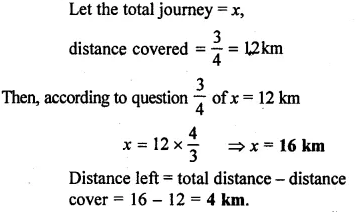Question 10.
When Ajit travelled 15 km, he found that one-fourth of his journey was still left. What was the full length of the journey?
Solution: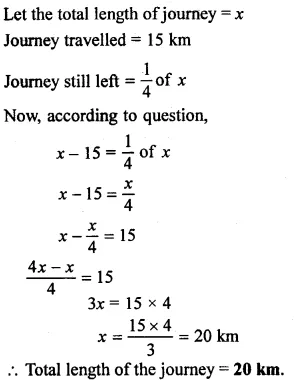Question 11.
In a particular month, a man earns Rs. 7,200. Out of this income, he spends $$\frac { 3 }{ 10 }$$ on food, $$\frac { 1 }{ 4 }$$ on house rent, $$\frac { 1 }{ 10 }$$ on insurance and $$\frac { 2 }{ 25 }$$ on holidays. How much did he save in that month ?
Solution: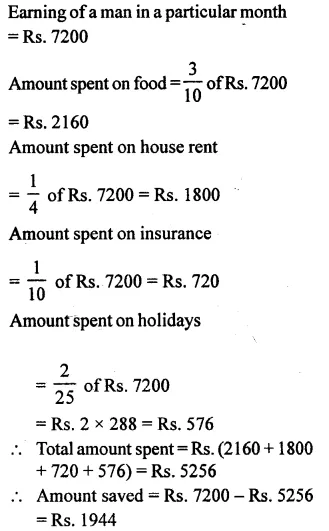### Fractions Revision Exercise – Selina Concise Mathematics Class 6 ICSE Solutions

Question 1.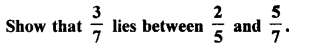Solution: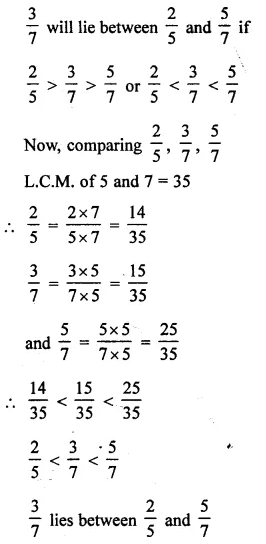Question 2.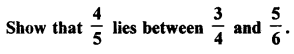Solution: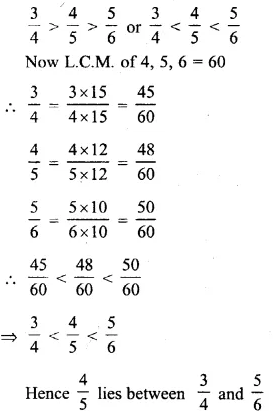Question 3.
Evaluate :Solution: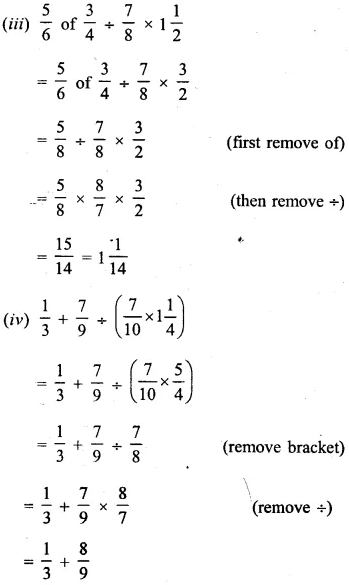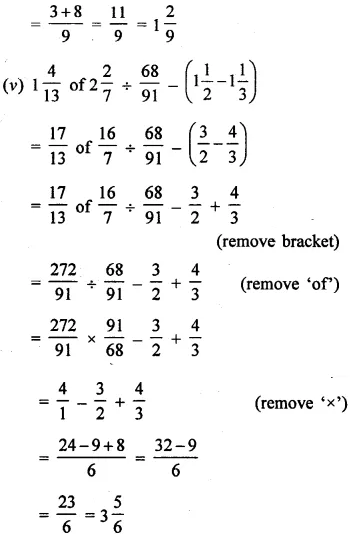Question 4.
Mr. Mehra gave one-third of his money to his son, one-fifth of his money to his daughter and the remaining amount to his wife. If his wife got Rs. 91,000, how much money did Mr. Mehra have originally?
Solution:Question 5.
A sum of Rs. 84,000 is divided among three persons A, B and C. If A gets one-fourth of it and B gets one-fifth of it; how much did C get?
Solution: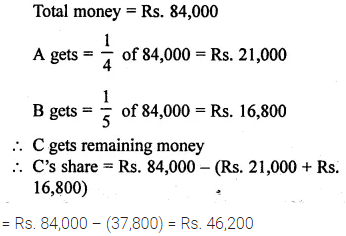Question 6.
In one hour Rohit walks 3$$\frac { 2 }{ 5 }$$ km. How much distance will he cover in 2$$\frac { 1 }{ 2 }$$ hours?
Solution: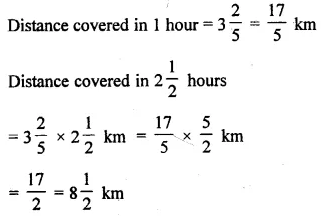Question 7.
An 84 m long string is cut into pieces each of length 5$$\frac { 1 }{ 4 }$$ m. How many pieces are obtained?
Solution: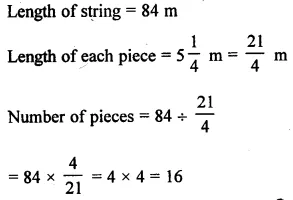Question 8.
In buying a ready made shirt-two-fifths of my pocket money is spent If Rs. 540 is still left with me, find :
(i) The money I had before I bought the shirt.
(ii) The emit of the shirt
Solution:Question 9.
Mohan leaves Rs. 1,20,000 to his wife and three children such that two-fifths of this money is given to his wife and the remaining is distributed equally among the children. Find, how much each child gets?
Solution: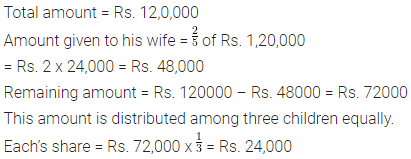Question 10.
Simplify :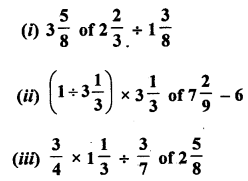Solution: